# Nested Qualifiers

Tom Kelliher, MA 190

Feb. 6, 2008

## Modeling Nested Qualifiers as Nested Loops

1.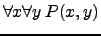Example: For every pair of real numbers, their sum is a real number.

proved := true;
for x := each element in its domain
confirmed := true;
for y := each element in its domain
if P(x, y) == false
confirmed := false;
break;

if confirmed == false
proved := false;
break;

return proved;


2.Example: For every integer,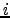, there is an integer,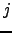, such that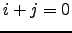.

proved := true;
for x := each element in its domain
confirmed := false;
for y := each element in its domain
if P(x, y) == true;
confirmed := true
break;

if confirmed == false
proved := false;
break;

return proved;


3.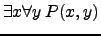Example: There is a real number that when multiplied by any real number,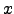, results in a product of.

proved := false;
for x := each element in its domain
confirmed := true;
for y := each element in its domain
if P(x, y) == false
confirmed := false;
break;

if confirmed == true
proved := true;
break;

return proved;


4.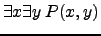Example: There are two integers whose product is 12.

proved := false;
for x := each element in its domain
confirmed := false;
for y := each element in its domain
if P(x, y) == true
confirmed := true;
break;

if confirmed == true
proved := true;
break;

return proved;


## Exercises

1. (1 a, b, c) Translate these statements into English. The domain is all real numbers.
1.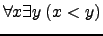2.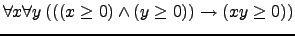3.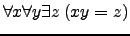2. (5 e) Translate this statement into English.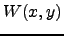means that studenthas visited web site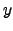. The domain foris all Goucher students and the domain foris all web sites.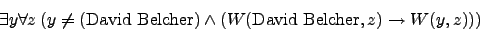3. (9 b, c, d, h) Let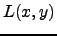be the statementloves,'' where the domain for bothandconsists of all people. Use quantifiers to express these statements.
1. Everybody loves somebody.

2. There is somebody whom everybody loves.

3. Nobody loves everybody.

4. There are exactly two people whom Lynn loves.

4. (37 b, c) Express each of these statements using quantifiers. Then, form the negation of the statement so that no negation is to the left of a quantifier. Next, express the negation in simple English.
1. Someone has visited every country in the world except Libya.

2. Every movie actor has either been in a movie with Kevin Bacon or has been in a movie with someone who has been in a movie with Kevin Bacon.

Thomas P. Kelliher 2008-02-02
Tom Kelliher